# 4. TMRA System

## 4. TMRA System

This section discusses the design and the detailed algorithm of the temporal multi-resolution analysis framework that can handle both CUT and GT transitions in a consistent manner. The major problem in shot boundary detection is the noise introduced by camera, object motion and flashes. We discuss the solution to eliminate the wrong boundaries and improve the system precision.

### 4.1 System Overview

The TMRA system has 3 phases. In the feature extraction phase, feature vectors suitable for the TMRA are computed. In the shot boundary detection phase, transitions are characterized and TMRA algorithm is applied to obtain the transition boundaries. This result will include many wrong transitions (insertions). In the elimination phase, motion analysis and flash detection are applied to remove insertions due to motion and abrupt flash noises. Figure 8.6 shows the system architecture for shot boundary detection. The following sections discuss in detail each of these three phases.Figure 8.6: Schematic of TMRA system for shot boundary detection.

### 4.2 Feature Extraction

In the current implementations, four popular feature representations for video streams are considered. To show that the framework can fit in uncompressed domain and compressed domain, the 64-bin color histogram and the 64-bin motion angle histogram are extracted in both domains. To show that the framework can fit into any well-defined feature space, the DC64 feature space is also used for the compressed domain.

###### 64-bin Color Histogram in Raw Domain

The color representation in the 64-bin color histogram feature space is in 6-bit coding, 2 bits for each color channel (R, G, B). Consider the color histogram h(n) for frame n denoted as h(n) = (b1,b2,,bn) where bi gives the ith color bin. The distance for frame i and frame j in this feature space (64-bin color histogram) is defined as:

 (8.15)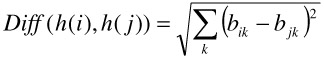###### 64-bin DCT DC Histogram from Compressed Domain -DC64

While it is straightforward to extract DC coefficients from I frame in MPEG video, motion compensation needs to be implemented in the DC extraction in P and B frames. The DC image is obtained after calculating the DC coefficients. In the system implementation, only the DC coefficients are used instead of DC+2AC . This is because processing speed is more important in compressed domain and AC is less important when calculating the global feature such as color histogram.

The DC image obtained in compressed domain can be used to form the DCHistogram feature for videos. In this feature space, the DCHistogram for every frame is denoted as DH(k), where DH(k) = (dcl1,dcl2,,dcl64), and the dclj represents the j th level of luminance. The distance between two frames in this feature space (DCHistogram) can be illustrated as

 (8.16)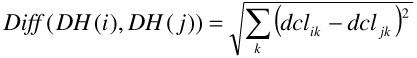Furthermore, we obtain the reduced DC image from DC image by dividing the original DC image into 8x8 bins, and computing the value of each bin as the average of the DC coefficients of all pixels covered by that bin. In this way, we reduce the original DC image to 64 bins, represented by: rdcl = (rp1, rp2,,rp64). Here rdcl denotes the reduced dc image for frame l, and rpm is the mth bin of the reduced DC image. It should be noted that each bin is attached to a specific location and thus this is a color-spatial representation.

The distance between two frames in the reduced DC feature space is:

 (8.17)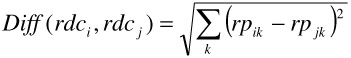###### 64-bin Motion Angle Histogram from Compressed Domain - MA64

The motion vector represents the motion of a macroblock of 16x16 pixels. It contains information about the type of temporal prediction and corresponding motion vectors used for motion compensation. A continuously strong inter-frame reference will be present in a stream as long as there is motion continuity. The temporal change in motion based feature vector can be used to define and characterize the occurrence of a transition. Significant instability of this feature is used as a sign of shot changes. So we incorporated a motion-based feature vector in our system along with the color based feature vector aiming to: (a) eliminate the falsely detected transitions caused by camera/object motion; and (b) accurately detect transition boundary. In MPEG compressed stream only forward motion vectors are used. In the case of B frames, averaging and approximations are applied to obtain forward motion vectors. Alternatively in the raw domain we compute the motion vectors using block based motion estimation such as spiral search method . Since the motion vectors tend to be sparse, a 3x3 median filtering is applied to the motion vectors. Also boundary blocks are not considered, as they tend to be erroneous. Motion angle is computed as

 (8.18)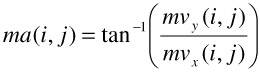where i, j represents macroblock row and column value.

The motion vectors obtained can be used to form the Motion Angle Histogram feature for videos. By quantizing the motion angles into intervals of 6 degrees, we can obtain a reduced MAHistogram consisting of 60 bins, represented by: rmal = (rp1, rp2,,rp60), where rpm is the mth bin of the reduced motion angle. It should be noted that each bin is attached to a specific location and thus this is a color-spatial representation.

The distance between two frames in the reduced motion feature space is:

 (8.19)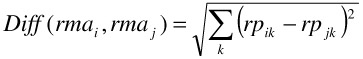To make our feature vector consistent with the DC64 feature space, we extended our 60-bin motion angle feature to a 64-bin feature space (MA64) by concatenating the counts of forward predicted macroblocks, backward predicted macroblocks, intra-coded macroblocks and skipped macroblocks. In the case of raw domain block based motion estimation only forward prediction is employed in which case we forced fc = bc = 0 which is not used in any of our computations for shot boundary detection. This feature space is very useful in identifying the transition boundaries and also in observing the motion activity.

### 4.3 Shot Boundary Detection

In this phase, TMRA algorithm is applied to determine the potential transition point and their type. It performs the wavelet transformation on the color and motion based feature vectors. The choice of feature vector is application specific and some examples are the HSV color based histogram, 256-bin intensity histogram, etc.

###### Transition Characterization

By making the fundamental observation that a video shot boundary is a multi-resolution phenomenon, video transitions can be characterized by the following features:

• Resolution of the transition;

• Strength of the transition;

• Length of the transition.

These features can be obtained respectively by:

• Counting the scale where the transition has been found; it is also the scaling parameter of the wavelet transform a in Equation 13.

• Computing the coefficients after the wavelet transformation as |Waf(x0)|, where x0 is the frame where the maxima is found in scale a.

• Computing the distance between start and end of transition.

In this way, all the transition points are represented in the whole framework by the three multi-resolution features.

###### TMRA Algorithm

Using the ideas in transition characterization, a temporal multi-resolution algorithm was developed to detect and characterize both CUT and GT shot boundaries. To illustrate the overall algorithm, we use a video named "Echosystem.mpg." This is an MPEG-1 compressed video sequence with 170 frames. The video sequence contains 2 CUT's and 3 GT' s. Sample sequence with frames 69–132 is shown in Figure 8.7. Figure 8.8 shows the frames following the 5 transitions. The overall algorithm is described as follows.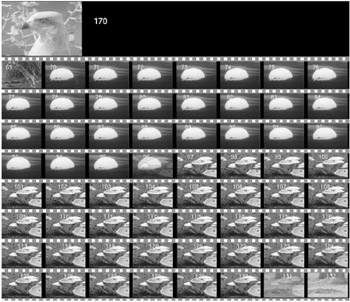Figure 8.7: Sample frames from the sequence Echosystem.mpg.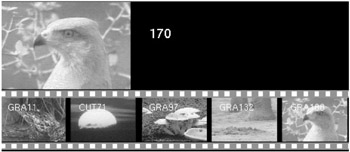Figure 8.8: Post transition frames of the video sequence Echosystem.mpg.

Table 8.1: Transition Boundary for Echosystem.mpg

Transition Type

Start Frame

End Frame

GT

9

11

CUT

70

71

GT

95

97

GT

130

132

CUT

159

160

1. Extract the feature vectors f(n) from the video stream

In the uncompressed domain, two feature vectors (DCHistogram and MA64) are obtained. DCHistogram is composed of the 64 color-histogram of every frame. MA64 is computed from the motion vectors computed from the block based motion estimation algorithm in raw domain.

In the compressed domain, three feature vectors are obtained in the model, DC64, DCHistogram and MA64. In the DC cases, DC coefficients are extracted first. The DCHistogram is obtained by counting the 64-bin luminance histogram of the DC image. The DC64 is obtained by splitting the DC image into 8 by 8 sub-windows and averaging every window to form the 64D feature vector. MA64 is computed from the extracted motion vectors from the compressed stream and along with the macroblock type counts.

1. Apply wavelet transformation on the video feature vectors

This is done by computing the Canny wavelet transform of f(x) for the temporal resolutions of a = 2j for j = 0 to 4. We thus obtain a set of coefficients of the form Wc = |Waf(x)|. By applying the transformation on the compressed domain DC64 and MA64 feature vectors, the corresponding wavelet coefficients obtained are WDC64 and WMA64, respectively. Figure 8.9 shows the WDC64 values of the video "Echosystem.mpg."Figure 8.9: Examples of coefficients after the transformation (WDC64).

1. Select the potential transition points at coarsest resolutions based on |Waf(x)| of DC64.

All local maxima are selected as potential transition points. The selection of local maxima is quite straightforward by tracing the feature vectors. When the feature vectors are changing from increasing to decreasing, the points are flagged as local maxima. This can be illustrated in Figure 8.10. In the illustration, the arrows indicate the trend of the signal, either increasing or decreasing, and the circles indicate the selected local maxima. Most of the local maxima in the higher temporal resolution space are either false or will be merged or transformed into a smaller number of local maximas in a coarser resolution space. And in the coarsest resolution space, only valid local maxima that correspond to transitions will be likely to remain. Thus for efficiency and effectiveness reasons, this step starts by selecting potential transition points in the coarsest resolution space.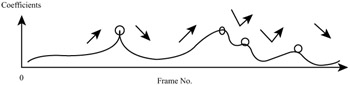Figure 8.10: Selecting the local maxima.

1. Find the 'start' and 'end' of each potential transition point using |Waf(x)| of MA64 at finest resolution.

The goal of video segmentation is not only to detect the occurrence of a transition, but also to locate the exact positions of the CUT/GT to segment the video. In a GT, both start position and end position need to be detected. Low resolution of WDC64 and WMA64 helps to detect the occurrence; while high-resolution helps to characterize the start and end of the transition.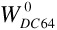represents high resolution RO and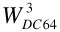represents coarse resolution R3, since gradual transition will have a slightly larger difference between frames than those within the normal shot. So in the higher resolution, the boundaries would show up as local maxima points. To identify this boundary, we use both the DC64 and MA64 wavelet coefficients. For coarse resolution (Resolution 3) DC64 wavelet coefficients are used and for high resolution (Resolution 0) MA64 wavelet coefficients are used. The reason for this choice is because DC64 feature space fails to characterize the beginning and ending frames of the transitions accurately at the high resolution. This is due to the fact that the rate of change in DC64 feature space is not high and doesn't result in a distinguishable peak. Hence we designed a new feature space based on the direction of motion along with counts representing static (skip) and intra (blocks having significant change) blocks. As a result of this we observe that even a gradual change resulted in an abrupt spike (peak) at the start and end of the transition. This is captured as the boundary of the transition.

For each potential transition point identified as local maxima in, we use it as the anchor point to search for the two nearest and strongest local maxima in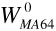. Thus for each potential transition we can identify the 'start' and the 'end' of the transition. This is illustrated in Figure 8.11. The two strong local maxima in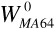are determined within the range of valley points enclosing the anchor point in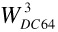. Two valley points enclose each potential transition point.Figure 8.11: Finding the start and end frame number of a transition.

1. Remove the potential transition points that correspond to noise by adaptive threshold

The problem of choosing an appropriate threshold is a key issue in applying TMRA for shot boundary detection. Heuristically chosen global threshold is not suitable as the shot content changes from scene to scene. So adaptive thresholds are better than a simple global threshold. The wavelet coefficient for DC64 will have many weak local maxima. These points should be deleted before the rest of the algorithm steps are applied. This will improve the performance of the system.

The adaptive threshold is determined by using a sliding window. The size of sliding window is a very important factor, which affects the final result directly. When the sliding window size is small, the threshold is low and more wrong transition points are selected. When the sliding window size is large, the threshold is higher, and thus may cause some correct transitions to be missed. By heuristics, we chose the sliding window size to be the sum of maximum distance between local maxima points and valley points. The threshold value is the average of local maxima points within the sliding window range. All local maxima below the adaptive threshold value are removed. This is illustrated in Figure 8.12.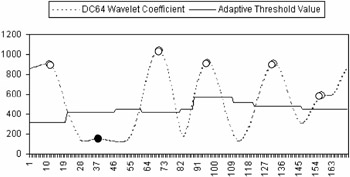Figure 8.12: Adaptive threshold for wavelet coefficients.

1. Classify transition typex

This is done by observing that the strength of potential transition points that correspond to noise tends to get severely attenuated at coarser resolutions, while those that correspond to CUT and GT will not be affected much. Thus by starting from coarse resolution space, only those genuine transitions remain. For each potential transition, we classify the transition points that correspond to CUT from those that correspond to GT by determining the distance between the 'start' and 'end' frames. For CUT, the frame difference is less than two and for GT it is more than two frames.

### 4.4 Elimination

The last phase of the TMRA algorithm is the elimination of wrong transitions due to motion and abrupt flashes in the shot. The following sections give a brief description on the method to characterize such activities in the multi-resolution framework.

###### Abrupt Flash Detection

Flash occurrences are common in video sequences, which often makes the shot detection method wrongly classify them as cut. In video segmentation system, flash detection is one of the difficult tasks that seriously affect the system performance. With the TMRA, this problem can be easily resolved by comparing WMA64 at different resolutions. Figure 8.13 shows an example where the strength of local maximas corresponding to a flash decreases drastically as the resolution decreases. This is because the duration of flash tends to be short. It thus results in large local maxima at higher resolution space, and attenuates quickly in lower resolution space. This can be extended to any other similar effects of flash, for example, the fire and fireworks, etc. By using this characteristic we can detect and remove such abrupt noises.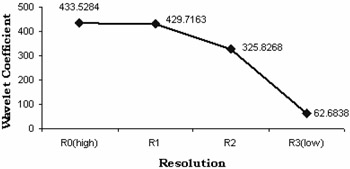Figure 8.13: Decreasing strength of WMA64 with lowering resolution corresponding to a flash.

###### Camera and Object Motion Detection

Fast camera and object motions account for a large number of wrong detection of transitions. Camera motion and object motion have the same effect as the gradual transition (for slow motion) and cut (fast motion). It is very difficult to distinguish them from correct transitions. With the TMRA framework, the MA64 feature vector is specially designed to capture the motion information.

As we expect the changes during a gradual transition to be relatively smooth, in principle, we expect the mean absolute differences (MAD) of DC64 and MA64 for the correct transitions (CUT/GT) to be consistent. Figure 8.14 illustrates the consistent and non-consistent portions of the mean absolute differences for DC64 and MA64 features. At CUT and GT points, MADs are consistent across DC64 and MA64. If the MAD changes are not consistent across DC64 and MA64, we conclude that it is a wrong transition caused by camera/object motion.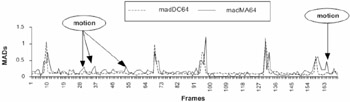Figure 8.14: Mean absolute differences for DC64 and MA64 features.

For each potential transition, the quadratic distance between mean absolute differences (QMAD) of the feature vector is computed. Three such quadratic MADs are computed between frames selected in the following manner.

 (8.20)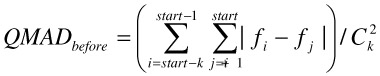(8.21)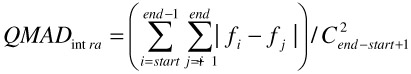(8.22)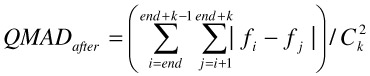where start and end represents the start and end frame number of potential transition. k is a scalar chosen to be between 4–9.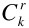is the normalizing factor in the above equations.

Here, QMADint ra is computed for the range of (start-1) to (end+1); MADbefore is computed based on the sequence of frames from (start-k) and start; and MADafter is computed based on the frame sequence end and (end+k)

The QMADs for the potential transitions (CUTs and GTs) are computed in both the DC64 and MA64 feature spaces. The following condition is checked to decide whether a transition is due to motion or is a real CUT/GT transition.

 (8.23)During a transition, the QMAD(dc) value within the transition tends to be more than the QMAD value in the before and after regions. The motion angle variation within the transition is minimal compared to the neighbouring before and after regions. By using this approach we can eliminate 70–80% of falsely detected transitions due to camera and object motions.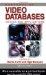Handbook of Video Databases: Design and Applications (Internet and Communications)
ISBN: 084937006X
EAN: 2147483647
Year: 2003
Pages: 393